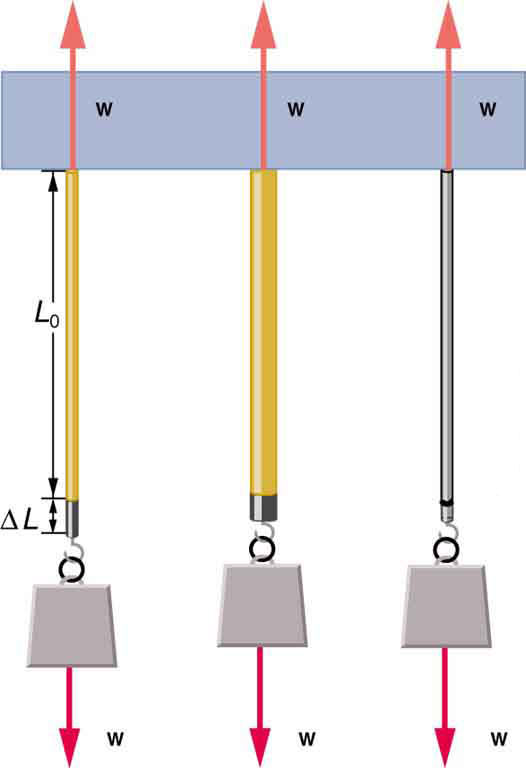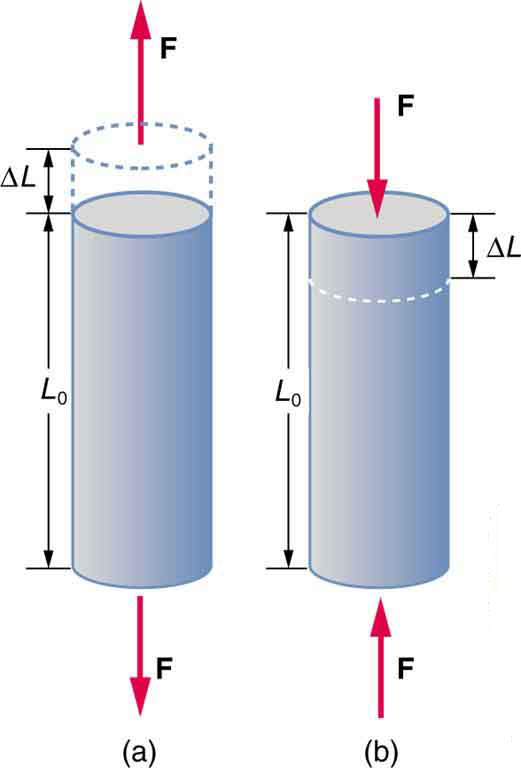# 5.3 Elasticity: stress and strain  (Page 2/15)

 Page 2 / 15The same force, in this case a weight ( w size 12{w} {} ), applied to three different guitar strings of identical length produces the three different deformations shown as shaded segments. The string on the left is thin nylon, the one in the middle is thicker nylon, and the one on the right is steel.

## Stretch yourself a little

How would you go about measuring the proportionality constant $k$ of a rubber band? If a rubber band stretched 3 cm when a 100-g mass was attached to it, then how much would it stretch if two similar rubber bands were attached to the same mass—even if put together in parallel or alternatively if tied together in series?

We now consider three specific types of deformations: changes in length (tension and compression), sideways shear (stress), and changes in volume. All deformations are assumed to be small unless otherwise stated.

## Changes in length—tension and compression: elastic modulus

A change in length $\Delta L$ is produced when a force is applied to a wire or rod parallel to its length ${L}_{0}$ , either stretching it (a tension) or compressing it. (See [link] .)(a) Tension. The rod is stretched a length Δ L size 12{ΔL} {} when a force is applied parallel to its length. (b) Compression. The same rod is compressed by forces with the same magnitude in the opposite direction. For very small deformations and uniform materials, Δ L size 12{ΔL} {} is approximately the same for the same magnitude of tension or compression. For larger deformations, the cross-sectional area changes as the rod is compressed or stretched.

Experiments have shown that the change in length ( $\Delta L$ ) depends on only a few variables. As already noted, $\Delta L$ is proportional to the force $F$ and depends on the substance from which the object is made. Additionally, the change in length is proportional to the original length ${L}_{0}$ and inversely proportional to the cross-sectional area of the wire or rod. For example, a long guitar string will stretch more than a short one, and a thick string will stretch less than a thin one. We can combine all these factors into one equation for $\Delta L$ :

$\Delta L=\frac{1}{Y}\frac{F}{A}{L}_{0},$

where $\Delta L$ is the change in length, $F$ the applied force, $Y$ is a factor, called the elastic modulus or Young’s modulus, that depends on the substance, $A$ is the cross-sectional area, and ${L}_{0}$ is the original length. [link] lists values of $Y$ for several materials—those with a large $Y$ are said to have a large tensile stifness because they deform less for a given tension or compression.

Elastic moduli Approximate and average values. Young’s moduli $Y$ for tension and compression sometimes differ but are averaged here. Bone has significantly different Young’s moduli for tension and compression.
Material Young’s modulus (tension–compression) Y $\left({\text{10}}^{\text{9}}\phantom{\rule{0.25em}{0ex}}{\text{N/m}}^{\text{2}}\right)$ Shear modulus S $\left({\text{10}}^{\text{9}}\phantom{\rule{0.25em}{0ex}}{\text{N/m}}^{\text{2}}\right)$ Bulk modulus B $\left({\text{10}}^{\text{9}}\phantom{\rule{0.25em}{0ex}}{\text{N/m}}^{\text{2}}\right)$
Aluminum 70 25 75
Bone – tension 16 80 8
Bone – compression 9
Brass 90 35 75
Brick 15
Concrete 20
Glass 70 20 30
Granite 45 20 45
Hair (human) 10
Hardwood 15 10
Iron, cast 100 40 90
Marble 60 20 70
Nylon 5
Polystyrene 3
Silk 6
Steel 210 80 130
Tendon 1
Acetone 0.7
Ethanol 0.9
Glycerin 4.5
Mercury 25
Water 2.2

Young’s moduli are not listed for liquids and gases in [link] because they cannot be stretched or compressed in only one direction. Note that there is an assumption that the object does not accelerate, so that there are actually two applied forces of magnitude $F$ acting in opposite directions. For example, the strings in [link] are being pulled down by a force of magnitude $w$ and held up by the ceiling, which also exerts a force of magnitude $w$ .

What is the difference between a principle and a law
the law is universally proved. The principal depends on certain conditions.
Dr
it states that mass of an element deposited during electrolysis is directly proportional to the quantity of electricity discharge
Olamide
what does the speedometer of a car measure ?
Car speedometer measures the rate of change of distance per unit time.
Moses
describe how a Michelson interferometer can be used to measure the index of refraction of a gas (including air)
using the law of reflection explain how powder takes the shine off a person's nose. what is the name of the optical effect?
WILLIAM
is higher resolution of microscope using red or blue light?.explain
WILLIAM
what is dimensional consistent
Mohammed
In engineering and science, dimensional analysis is the analysis of the relationships between different physical quantities by identifying their base quantities and units of measure and tracking these dimensions as calculations or comparisons are performed
syed
can sound wave in air be polarized?
Unlike transverse waves such as electromagnetic waves, longitudinal waves such as sound waves cannot be polarized. ... Since sound waves vibrate along their direction of propagation, they cannot be polarized
Astronomy
A proton moves at 7.50×107m/s perpendicular to a magnetic field. The field causes the proton to travel in a circular path of radius 0.800 m. What is the field strength?
derived dimenionsal formula
what is the difference between mass and weight
assume that a boy was born when his father was eighteen years.if the boy is thirteen years old now, how is his father in
Isru
31yrs
Olamide
what is airflow
derivative of first differential equation
why static friction is greater than Kinetic friction
draw magnetic field pattern for two wire carrying current in the same direction
An American traveler in New Zealand carries a transformer to convert New Zealand’s standard 240 V to 120 V so that she can use some small appliances on her trip.
What is the ratio of turns in the primary and secondary coils of her transformer?
nkombo
what is energy
Yusuf
How electric lines and equipotential surface are mutually perpendicular?
The potential difference between any two points on the surface is zero that implies È.Ŕ=0, Where R is the distance between two different points &E= Electric field intensity. From which we have cos þ =0, where þ is the angle between the directions of field and distance line, as E andR are zero. Thus
sorry..E and R are non zero...

#### Get Jobilize Job Search Mobile App in your pocket Now!ByBy Anindyo MukhopadhyayBy OpenStaxBy Qqq QqqBy Anh DaoBy OpenStaxBy Brooke DelaneyBy Vongkol HENGBy Stephen VoronBy Joli JuliannaBy Ann Schlosser